Applications to the mechanical models, acoustics and geophysics   Although our method is young, its applicability to the widest range of practical investigations is already obvious. It can be easy extended to the torsion vibrations; instead the longitudinal and transversal co-ordinates one can introduce those generalised; and so on. Even not transiting to the spatial problems, these solutions can be applied to 1D processes in elastic rods. In Fig. 14 we show the pattern of transversal vibrations in a semi-finite rod, which accompany those longitudinal and propagate along the rod lateral surface. However these waves are not Rayleigh waves, though the production of these last is caused also by the material transversal deformation under longitudinal vibrations. Their propagation velocity is equal to that of longitudinal waves and is not determined by Poisson coefficient, as Rayleigh waves are. The more, we can prove that if the propagation velocity of transversal waves accompanying the longitudinal waves differed from these last, then in the elastic line there occurs a paradoxical phenomenon: in the region of rod local tension its cross-section increases, and with compression it tapers.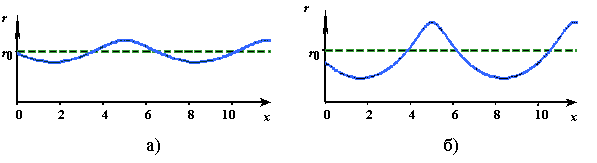Fig. 14. General form of the transversal surface wave propagating in a semi-finite elastic rod having finite cross-section, under the longitudinal dynamical load on the free end of the rod whose value is 0,2 of that critical (a) and 0,5 of critical (b) In the presented plots one can see one more feature of this process. At small external force amplitudes the surface waves are practically sinusoidal, and it can be corroborated mathematically. With the growing external force amplitude the wave deforms, and at its definite value in the region of dynamical compression the rod breakage occurs (the vibration amplitude turns into infinity). With it we investigated all vibration pattern transformations in frames of linear modelling, but with the help of our complete, determined, non-matrix analytical solutions.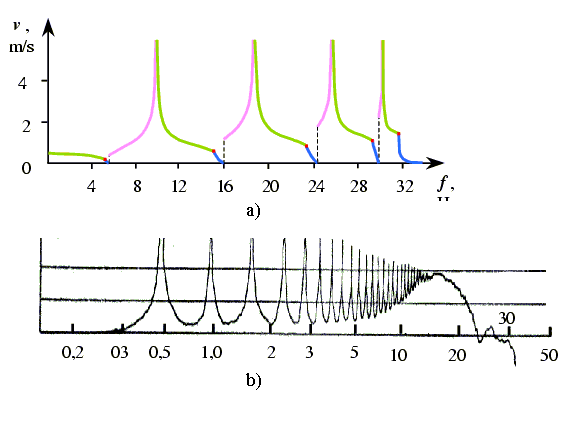Fig. 15. The amplitude-frequency characteristic of vibration velocity of the first element of a semi-finite elastic line having the resonance subsystems (a) and the experimental regularity for the longitudinal wave propagation velocity in a polyethylene rod as a function of frequency (b) In Fig. 15  we show two plots. In the left plot one can see the amplitude-frequency characteristic for an elastic system having the resonance subsystems obtained by our method, and in the right plot � the experimental data by Skudrzyk [17, p.244]. With the exception that the solutions did not take into account the line resistance, while the experimental data were obtained on the material having a resistance, the form of resonance peaks coincides. The difference is only in the splashes at the bottom of the left plot which correspond to the aperiodical vibration regime and, as we already know, will smooth with the growing resistance. As to vibration systems having the resonance subsystems, we underline again an important aspect that the resonances in these models arise just at the border between the critical frequency for the system as a whole and that for the subsystem. The answer to this question consists of a whole complex of answers to many questions of the science and practice. For example, an atom also is a vibration system having the resonance subsystems. And an orbiting electron does not radiate, but when transiting into another orbit at the subcritical frequency, it radiates. We can present alike instants in more practical areas, such as mechanical, turbine or aircraft engineering. Aren�t there reflected the errors by the engineers designing the mechanisms loaded by high-frequency vibrations? One knows, the problems of conventional methods arise just on high frequencies near the critical frequency.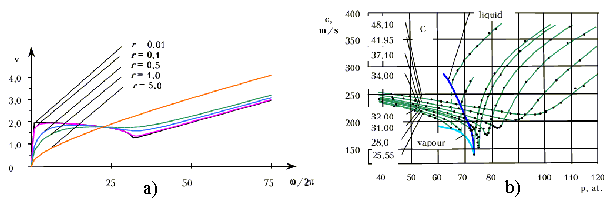Fig. 16. The phase velocity of wave propagation vp in a resistant elastic line as a function of frequency (a) and the experimental data for the propagation velocity c of ultrasonic vibrations as a function of pressure p in the vicinity of gas � vapour critical point for the carbonic acid gas (b) In Fig. 16  we show two plots in the acoustic area. In the left plot we present again the regularity of phase velocity of the wave propagation in a resistant elastic line. In the right plot we show the experimental result on the ultrasonic vibration propagation in the carbonic acid gas near the vapour/gas critical point against the pressure and temperature after [23, p.45]. Comparing the obtained theoretical results with the experimental regularity, we see that their patterns completely coincide. The growing velocity in Fig. 16b and the minimums smoothing, the same as the minimums displacement with the growing temperature, are fully described by the pattern of the parameters variation in the analytical regularities on whose basis Fig. 16b has been plotted. Each regularitiy c(p) in Fig. 16b has its typical minimum at the critical frequency. With growing temperature this minimum displaces towards the higher pressure (or higher frequency), and the sharpness of minimum smoothes because of line resistance growing. At the same time the wave propagation velocity increases because of intermolecular distance increasing. Speaking of application of the solutions obtained by the non-matrix method, we would like to mention briefly the geophysical investigations. It is commonly known that �almost all seismic zones � are located on the geosynclines and are inherent in them� [39, p.303], and �the earthquakes epicentres are located along the largest cracks, or these cracks are the epicentres themselves. In the tablelands the seismic phenomena are much uncommon� The cracks related to the kinks of wreath-likely placed folded mountains are especially liable to the earthquakes, and this coincidence is specifically inherent in tertiary mountain ranges surrounding Asia. More often than elsewhere on the Earth the seismic motions reveal in the Japan Great Graben. Almost all Indian earthquakes are concentrated in the corners where the Himalayan Arc meets that Iranian from one side and the Malay from another. Unfortunately, the sufficient attention still was not given to the close relation between the lines of folds meeting and the shock lines. But this dependence is observed in the ancient folded areas� [39, pp.301 � 303]. Even �the degree of shores seismic ability corresponds to the degree of steepness of their slopes� [39, p.304]. Noting that in most of rocks the transversal stiffness relates to that longitudinal as 1,5 � 2 [40, pp. 56 � 61], it is sufficient to see Fig. 17 (the copy of Fig. 12 of The main results of our method in brief made here for your convenience) to grasp the reason of this phenomenon.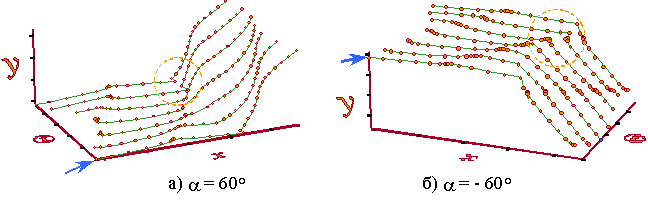Fig. 17. The typical vibration diagrams in a homogeneous elastic line with the positive (a) and negative (b) kink angles under the inclined external force action and with the inequal longitudinal and transversal stiffness coefficients, as the function of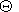Actually, a few examples presented here cannot cover the entire spectrum of the applications. However they also demonstrate quite visually, how complex and important solutions can the practice obtain with the help of the advantages the new non-matrix method to obtain exact analytical solutions gives.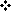ProposalApplications to the mechanics, acoustics and geophysicsApplications to the mismatched ladder filters, transmission lines and networks